EBSD Orientation Anaylsis edit page

Here we discuss tools for the analysis of EBSD data which are independent of its spatial coordinates. For spatial analysis, we refer to this page. Let us first import some EBSD data: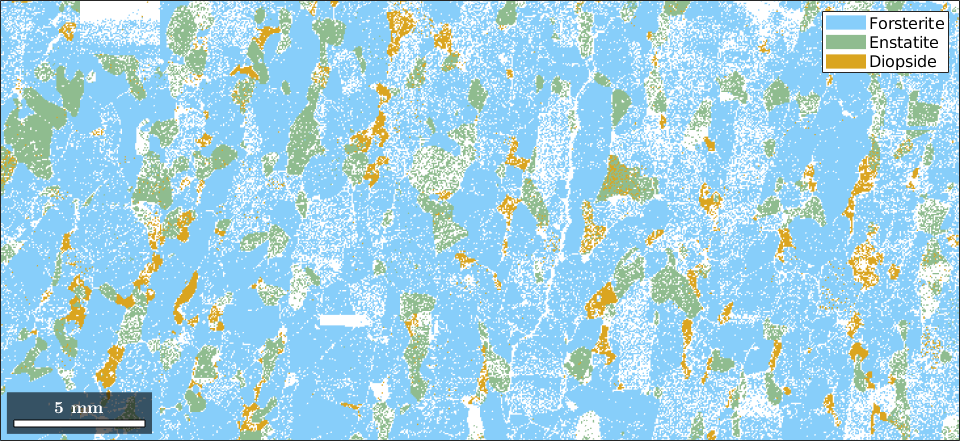## Orientation plot

We start our investigations of the Forsterite phase by plotting some pole figures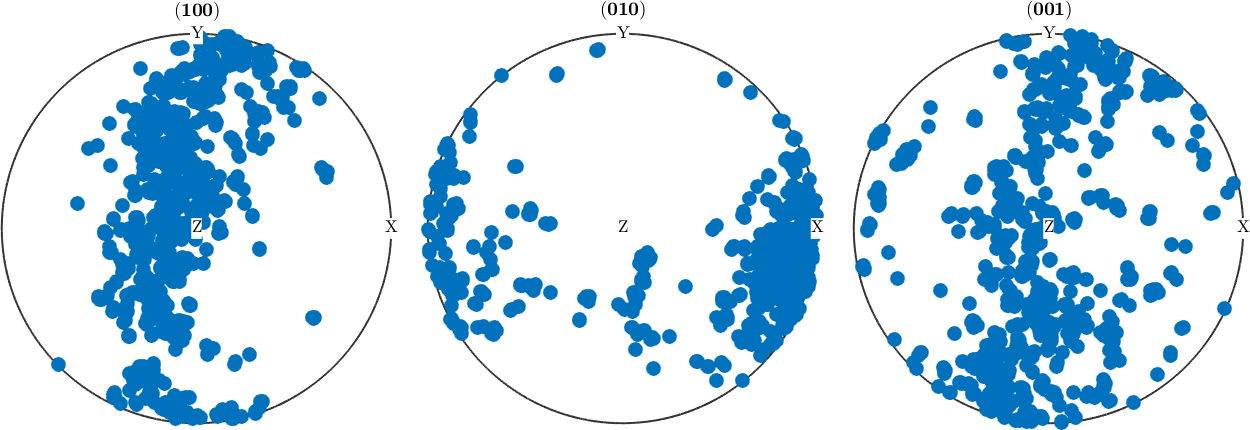From the {100} pole figure, we might suspect a fibre texture present in our data. Let's check this. First, we determine the vector orthogonal to fibre in the {100} pole figure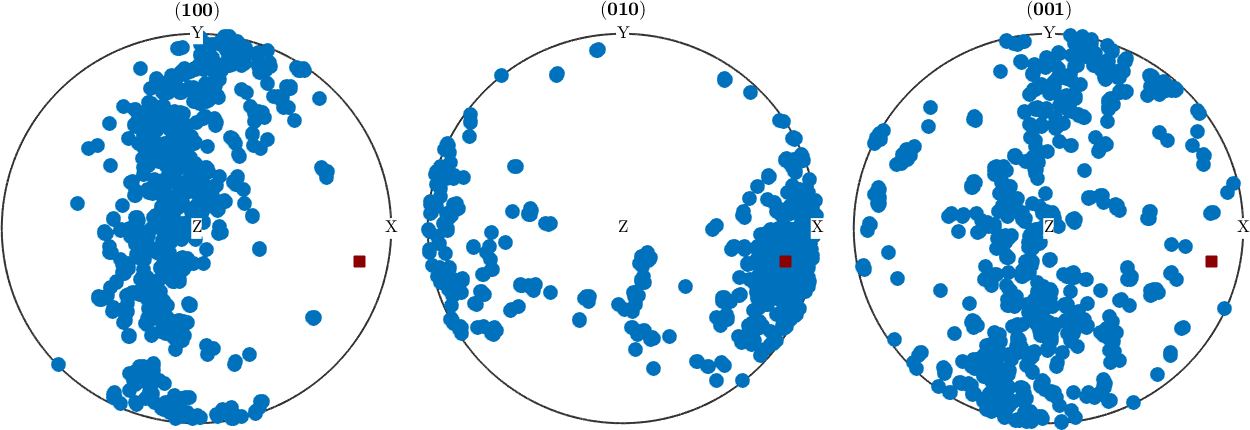we can check how large is the number of orientations that are in the (100) polegigure within a 10-degree fibre around the great circle with center rOrth. The following line gives the result in percent

Next, we want to answer the question which crystal direction is mapped to rOrth. To this end, we look at the corresponding inverse pole figure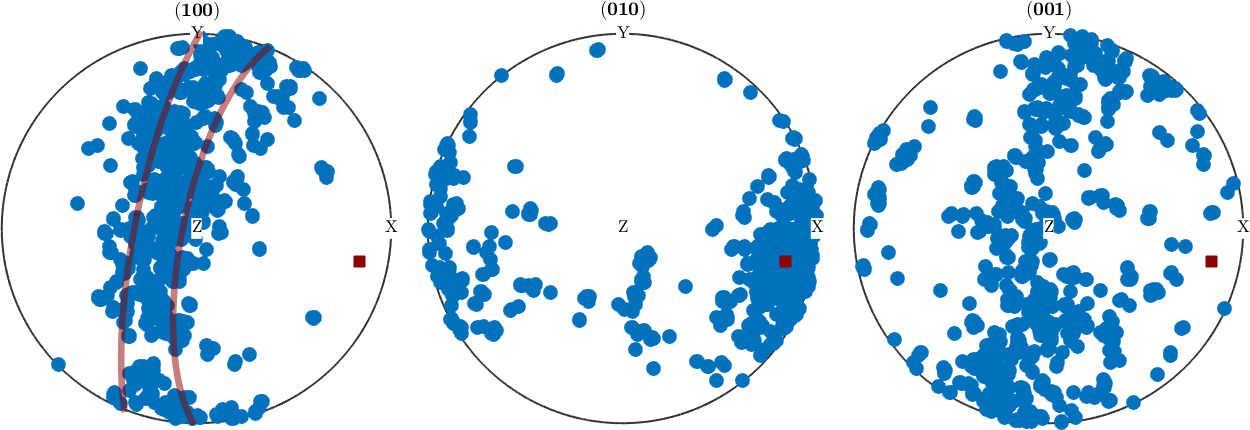Surprisingly this value is significantly lower than the value we obtained we looking only at the 100 pole figure. Finally, let's plot the ODF along this fibre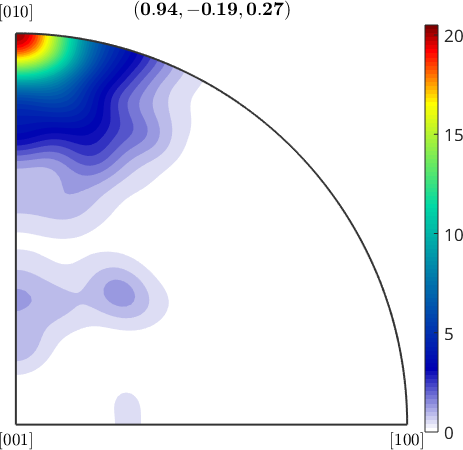We see that to ODF is far from beeing constant along the fibre. Thus, together with an observation about the small fibre volume, we would reject the hypothesis of a fibre texture.

Let's finally plot the ODF in orientation space to verify our decision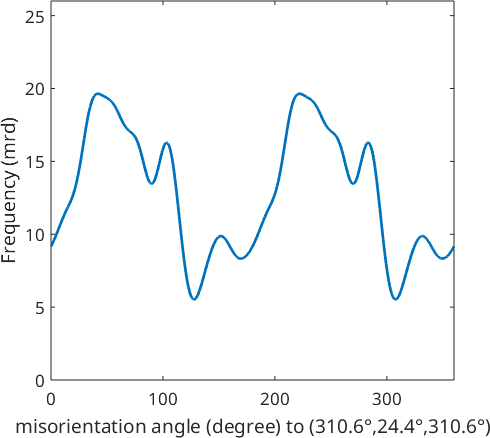Here we see the typical large individual spots that are typical for large grains. Thus the ODF estimated from the EBSD data and all our previous analysis suffer from the fact that too few grains have been measured. For texture analysis, it would have been favorable to measure at a lower resolution but a larger region.